# Centerless implies inner automorphism group is centralizer-free in automorphism group

## Statement

Suppose$G$ is a Centerless group (?): the center of$G$ is trivial. Then, the Inner automorphism group (?)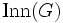$\operatorname{Inn}(G)$ (which is naturally isomorphic to$G$) is a Centralizer-free subgroup (?) inside$\operatorname{Aut}(G)$: there is no non-identity automorphism of$G$ that commutes with every inner automorphism.

Equivalently, under the identification of$G$ with$\operatorname{Inn}(G)$, we say that$G$ is centralizer-free in$\operatorname{Aut}(G)$. Note that this also implies that$\operatorname{Aut}(G)$ is a centerless group.

## Facts used

1. Group acts as automorphisms by conjugation: In particular,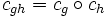$c_{gh} = c_g \circ c_h$, where$c_g$ is the map$x \mapsto gxg^{-1}$.

## Proof

### Hands-on proof

Given: A group$G$, with automorphism group$\operatorname{Aut}(G)$ and inner automorphism group$\operatorname{Inn}(G)$. Here, for$g \in G$, the inner automorphism$c_g$ is defined as$x \mapsto gxg^{-1}$.

To prove: If$\sigma \in \operatorname{Aut}(G)$ commutes with$c_g$ for all$g \in G$, then$\sigma$ is the identity map.

Proof:

1. For all$g \in G$,$\sigma \circ c_g \circ \sigma^{-1} = c_{\sigma(g)}$: For any$x \in G$, the left side is$\sigma(g\sigma^{-1}(x)g^{-1}) = \sigma(g)\sigma(\sigma^{-1}(x))\sigma(g)^{-1} = \sigma(g)x\sigma(g)^{-1} = c_{\sigma(g)}(x)$. Thus, the two sides are equal for all$x \in G$, and are hence equal as functions.
2. If$\sigma$ and$c_g$ commute, we have$c_g = c_{\sigma(g)}$: This follows directly from step (1).
3. If$\sigma$ and$c_g$ commute, we have that$c_{g^{-1}\sigma(g)}$ is the identity map: Since$c_g = c_{\sigma(g)}$, we get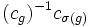$(c_g)^{-1}c_{\sigma(g)}$ is the identity map. Using the fact that conjugation is a group action, we obtain that$c_{g^{-1}\sigma(g)}$ is the identity map.
4. (Given data used:$G$ is centerless): If$\sigma$ and$c_g$ commute,$\sigma(g) = g$: Step (3) shows that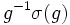$g^{-1}\sigma(g)$ acts trivially by conjugation, and is hence in the center. Since we know that$G$ is centerless,$g^{-1}\sigma(g)$ must be the identity element, yielding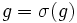$g = \sigma(g)$.
5. If$\sigma$ commutes with$c_g$ for every$g \in G$,$\sigma$ is the identity map: This is a direct consequence of step (4), applied to all$g \in G$.

### Proof using the commutator language

Given: A group$G$, with automorphism group$\operatorname{Aut}(G)$ and inner automorphism group$\operatorname{Inn}(G)$. Here, for$g \in G$, the inner automorphism$c_g$ is defined as$x \mapsto gxg^{-1}$.

To prove: If$\sigma \in \operatorname{Aut}(G)$ commutes with$c_g$ for all$g \in G$, then$\sigma$ is the identity map.

Proof: For convenience, we identify$G$ with the subgroup$\operatorname{Inn}(G)$ of$\operatorname{Aut}(G)$. Note that$[G,\sigma]$ now has two interpretations:

• It is the subgroup of$\operatorname{Aut}(G)$ generated by commutators between elements of$G$ and the automorphism$\sigma$, viewed as automorphisms of$G$.
• It is the subgroup of$G$ generated by elements of the form$g^{-1}\sigma(g)$, where$g \in G$.

The former interpretation tells us that$[G,\sigma]$ is trivial, which, viewed using the latter interpretation, yields that$\sigma$ is the identity automorphism.